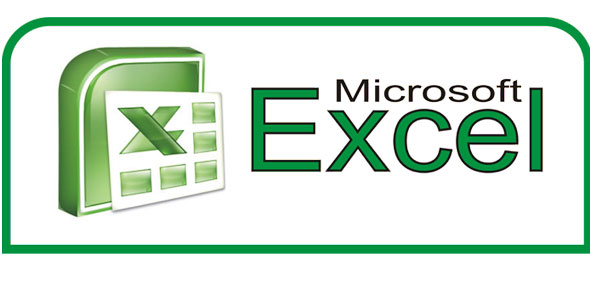# Microsoft Office 2013: Excel 101 Quiz!

10 Questions | Total Attempts: 26888SettingsWelcome to this short yet important Microsoft Office 2013 quiz on Excel. Here, you will be asked ten fundamental questions of this application just to test your basic understanding of the same. Let's see if you can pass all the questions without getting a single one wrong or not.

Related Topics
• 1.
The first cell in EXCEL worksheet is labeled as:
• A.

AA

• B.

A1

• C.

Aa

• D.

A0

• 2.
• A.

An absolute cell address is created.

• B.

Cell address will change when it is copied to another cell.

• C.

The sheet tab is changed.

• D.

The status bar does not display the cell address.

• 3.
What are the tabs that appear at the bottom of each workbook called?
• A.

Reference tabs

• B.

Position tabs

• C.

Location tabs

• D.

Sheet tabs

• 4.
What is represented by the small, black square in the lower-right corner of an active cell or range?
• A.

Copy handle

• B.

Fill handle

• C.

Insert handle

• D.

Border

• 5.
In Excel, a Data Series is defined as what?
• A.

A type of chart

• B.

A cell reference

• C.

A collection of related data

• D.

A division of results

• 6.
In Excel, the Fill Color button on the Formatting toolbar is used for what?
• A.

To insert a background.

• B.

• C.

To select a distribution of figures.

• D.

• 7.
In help menu of Excel, which of the following tabs are found?
• A.

Contents tab

• B.

• C.

Index tab

• D.

All of the above

• 8.
A __________ is a grid with labeled columns and rows.
• A.

Dialog box

• B.

Worksheet

• C.

Clipboard

• D.

Toolbar

• 9.
The active cell:
• A.

Is defined by a bold border around the cell

• B.

• C.

It is the formula bar

• D.

Receives data user enters & defined by a bold border around the cell

• 10.
Which function is used to calculate depreciation, rates of return, future values and loan payment amounts?
• A.

Logical

• B.

Math & Trigonometry

• C.

Statistical

• D.

Financial